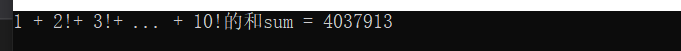• C语言编写10的阶乘,用C语言编程求10的阶乘C语言编写10的阶乘,用C语言编程求10的阶乘匿名网友:思路：先定义一个函数求一个数的阶乘，接着依次从1到10调用该函数就1到10的阶乘并输出。参考代码：#include<stdio.h&...
导航：网站首页 >C语言编写10的阶乘,用C语言编程求10的阶乘C语言编写10的阶乘,用C语言编程求10的阶乘匿名网友:思路：先定义一个函数求一个数的阶乘，接着依次从1到10调用该函数就1到10的阶乘并输出。参考代码：#include<stdio.h>int fun(int n){//定义函数求n的阶乘 if(n==0||n==1) return 1;else return n*fun(n-1);} int main(){int i;for(i=1;i<=10;i++)printf(＂%d的阶乘为：%d\n＂,i,fun(i));return 0;} /*输出：1的阶乘为：12的阶乘为：23的阶乘为：64的阶乘为：245的阶乘为：1206的阶乘为：7207的阶乘为：50408的阶乘为：403209的阶乘为：36288010的阶乘为：3628800*/匿名网友:#include<stdio.h>int fac(int n)； //函数声明void main(){int a;//int m;printf(＂input a int number:＂);scanf(＂%d＂,&a);printf(＂after the function is:＂);//m=f(a);printf(＂%d\n＂,fac(a));}int fac(int n) //定义fac函数{if(n<0){printf(＂n<0,date error!＂);}elseif(n==0||n==1){return 1;}elsereturn (n*fac(n-1));}注意输入的数不能太大了，否则就会出现溢出的情形。比方说10000的阶乘就要用数组来实现了，这个程序是无法办到的。匿名网友:/*4037913Press any key to continue*/#includelong fact(int n) {int i;long fac = 1;for(i = 2; i return fac;}void main() {int i;long sum = 0;for(i = 1; i sum += fact(i);printf(＂%ld\n＂,sum);getchar();}匿名网友:★172666706＂>int fun(int n) /*求n的阶乘*/ { if(n > 1) return n*fun(n - 1); if(n == 1) return 1; } 1.do while 语句 int sum = 0; int i = 1; do { sum += fun(i); i++; }while(i <= 10) 2.while语句 int sum = 0; int i = 1; while(i <= 10) { sum += fun(i); i++; }问题推荐
展开全文• =10)，实现m!/n!/(m-n)!。 注：以下列出0至5的阶乘： 0！=1，注意(0的阶乘是存在的) 1！=1， 2！=2， 3！=6， 4！=24， 5！=120， 输入 每行输入一组测试数据：2个正整数m和n。m与n均为非负整数。 m和n均为0时，...
题目描述：编写一求x!的函数（0<=x<=10)，实现求m!/n!/(m-n)!。
注：以下列出0至5的阶乘：
0！=1，注意(0的阶乘是存在的)
1！=1，
2！=2，
3！=6，
4！=24，
5！=120，
输入
每行输入一组测试数据：2个正整数m和n。m与n均为非负整数。
m和n均为0时，结束输入。
输出
输出m!/n!/(m-n)!的计算结果，结果或为单精度浮点型数据，或为错误（“Error”）。
样例输入
11,10
10,9
5,2
5,0
0,5
5,5
0,0
样例输出
Error
10.000000
10.000000
1.000000
Error
1.000000
#include <stdio.h>
float fuc(int i)
{
int p;
if(i==1||i==0) p=1;
else  p=i*fuc(i-1);
return p;
}

int main()
{
int n,m,x,y,z;
float sub;
while (scanf("%d,%d",&n,&m)!=EOF&&m+n!=0)
{
if(m>10||n>10) printf("Error\n");
else
if(n-m<0) printf("Error\n");
else
{
x=fuc(n); y=fuc(m); z=fuc(n-m);
sub=x/y/z;
printf("%f\n",sub);
}
}
return 0;
}




展开全文• 1 + 2!+ 3!+ … + 10!和 #include<stdio.h> int main() { int sum = 0; int n = 1; for (int i = 1; i <= 10; i++) { n = n * i; sum = sum + n; } printf("1 + 2!+ 3!+ ... + 10!和sum = %d...
求1 + 2!+ 3!+ … + 10!的和
#include<stdio.h>
int main()
{
int sum = 0;
int n = 1;
for (int i = 1; i <= 10; i++)
{
n = n  * i;
sum = sum + n;
}
printf("1 + 2!+ 3!+ ... + 10!的和sum = %d\n", sum);
return 0;
}展开全文• 的阶乘增长非常快速，传统的数据无法满足计算需求，需要用一个数组存放数据。#include #define lim 10int n1;int top;void cout();long m1,m2;void multiply(int t){ int i=0,j=0,k; while(1) { n1[i]*...
数的阶乘增长非常快速，传统的数据无法满足计算需求，需要用一个数组存放数据。
#include <stdio.h>#define lim  10int n1;int top;void cout();long m1,m2;void multiply(int t){    int i=0,j=0,k;   while(1)   {      n1[i]*=t;      n1[i]=n1[i]+j;      if(n1[i]>=lim)      {j=n1[i]/10;n1[i]=n1[i]%10;}      else      {          j=0;      }      i++;      if(i>top)        break;   }       while(j!=0)       {           n1[i++]=j%10;           j=j/10;       }       top=i-1;}void final(){   int i,t;   t=0;   for(i=0;i<=top;i++)   {       n1[i]+=t;      if(n1[i]>10)      {          t=n1[i]/10;          n1[i]=n1[i]%10;      }      else        t=0;   }   while(t!=0)   {      n1[i++]=t%10;      t=t/10;   }   top=i-1;}void cout(){    int i=0;    printf("result:/ttop:%d/t",top);    for(i=top;i>=0;i--)    {        printf("%d",n1[i]);    }    for(i=0;i<=top;i++)    {        m2=m2*10+n1[i];    }    printf("/n");}void factorial(int t){   int i,j,k,m;   printf("factorial:%d/n",t);   k=0;   m=t;   while(1)   {      i=t%10;      n1[k]=i;      if(t=t/10)        k++;      else        break;   }   top=k;
for(i=m-1;i>=2;i--)    multiply(i);
final();   cout();    }int main(){    factorial(400);    getch();    return 0;}
1000位的数组可以实现400的阶层，有868位
如果不惜空间而吝惜时间，那么lim可以设置的大一点，可以减少运算次数
展开全文语言 c
•  //阶乘的任一元素与临时结果某位乘积结果  int i, j, carry; //carry：进位  printf("please in put n:\n");  scanf("%d",&n);  a = 1; //将结果先初始化为1  for ( i=2; i; i++ ) //开始阶乘，...c 算法
• *单链表结点的阶乘和 *2019-10-17 *qq_2967953656 */ #include <stdio.h> #include <stdlib.h> //单链表的的定义 //定义了一个指向Node类型的指针别名 typedef struct Node *PtrToNode; //...
• 某书上写程序太复杂，还要区分0和1情况，其实没必要。 废话不多说，上代码： #include <stdio.h> void main() { int m = 1;...=10; i++){ m = m * i; } printf("%d\n", m); } ...
• 1.将递归求阶乘封装函数 2.1！-10和 代码实现： #include<stdio.h> int jiecheng(int n){ if(n==0){ //递归正常结束 return 1; } return n*jiecheng(n-1);//递归调用 } int main(){ int n=0; ...递归法
• C语言 - 求阶乘和 编程S=1！+2！+3！+…+N！值(N<=20)。 Example Input 10 Example Output 4037913 代码： #include<stdio.h> int main(){ int input,i,j; long sum=1,output=0; printf("Example ...算法
• 如何在C语言中计算大数阶乘发布时间：2021-05-10 15:54:36来源：亿速云阅读：59作者：Leah今天就跟大家聊聊有关如何在C语言中计算大数阶乘，可能很多人都不太了解，为了让大家更加了解，小编给大家总结了以下内容，...
• 输入一个正整数n,n!(即阶乘)末尾有多少个0？ 比如: n = 10; n! = 3628800,所以答案为2    输入描述: ...输入为一行，n(1 ≤ n ≤ 1000) ...输出一个整数,即题目所 ...阶乘末尾后个数 */ ...
• ## C语言——求1到10的阶乘之和

万次阅读 多人点赞 2019-05-11 23:58:01
#include<stdio.h> int main() { double a,b,c,sum=0; for(a=1;a<=10;a++) { for(b=1,c=1;b<=a;b++) { c=c*b; } sum = sum + c;...同理，如果1到一个已知整数Number的阶乘，只需做以下修改与替...for循环
• //计算 一到十 阶乘的和 #include <stdio.h> void main() { int i=1; int j=1; int sum=1; double sum1=0; while(i<=10) { while(j<=i) { sum=sum*j; j++; ...
• 循环法 循环法还是比较简单的，就先输入你要求...//10 以上的阶乘就比较大了 int i; printf("请输入你要求的阶乘：") scanf("%d",&n);//先输入要求的数 for(i=n;i>0;i--)//乘到1为止 { sum*=i; ...
• 6.17C语言1~10的阶乘之和 #include<stdio.h> int main(){ int n; long sum=0,f=1; for(n=1;n<=10;n++){//循环穷举n的取值 f*=n; //迭代公式，累乘计算n! sum+=f; //累加求和 } printf...
• 下面小编就为大家带来一篇C语言练习题:1到10的阶乘之和简单实例。小编觉得挺不错的，现在分享给大家，也给大家做个参考。一起跟随小编过来看看吧
• C语言大数阶乘运算答案:4信息版本：手机版解决时间 2019-10-06 23:22已解决2019-10-06 05:26一份计算大数阶乘的代码 从1！一直算到40！不需要相加 输出时候 是1! = %d\n 2! = %d\n …… 40! = %d\n 每一位数用一...
• 这篇文章主要给各位讲述C语言实现N阶乘的程序代码，所谓n的阶乘就是从1到n的累积,所以可以通过一个for循环,从1到n依次积即可，想详细了解的朋友赶紧跟随爱站技术频道的小编一起往下学习吧。代码如下所示：复制代码...
• #include<stdio.h> int main() { int i,k; long s=1,sum=0; for(i=1;i<=10;i++) { s*=i; printf("%d的阶乘为%ld\n",i,s);...printf("1到10的阶乘之和为：%ld\n",sum); return 0; } ...
• C语言---n的阶乘后面有多少个连续的0 题目描述：给定一个正整数n，返回n的阶乘尾部连续0的个数。 例如：（5，5*4*3*2*1=120，则返回1），（1010*9*8*7*6*5*4*3*2*1=3628800，则返回2） 题目分析：首先拿到...
• 从键盘输入1~10整数n，输出1!+2!+3!+…+n!值，或者输出"input error" 输入样例：4 输出样例：33 //该代码使用Microsoft VS2019编写 #define _CRT_SECURE_NO_WARNINGS #include<stdio.h> #include<...
• 当你的能力匹配不了你的野心时候,是该静下心来提深自己!...小编以求10的阶乘为例，给大家分享下阶乘的求法： #include &lt;stdio.h&gt; int prime(int x); void main(){ int m,n; prin...递归
• //1的阶乘 //计算阶乘 //*********这一段计算阶乘不懂啊*********** for(i = 2; i ; i++) { for(j = 0; j ; j++) { s = a[j] * i + c; a[j] = s % 10; c = s / 10; } } //去掉前面为0的 ...c++ 开发语言
• 1-10的阶乘 #include"stdio.h" void main() { long a=1,n,s=0; for(n=1;n<=10;n++) { a*=n;/*阶乘*/...

# c语言求10的阶乘c语言 订阅